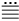SEARCH HOMEMath Central Quandaries & QueriesQuestion from Randy, a parent: We all know how to count (in the base 10/decimal system) using words not numbers. For example: one, two, three, four, five, six, seven, eight, etc. However, in base 5 (for example) how would you count (in words). For sure - in base 5 the number 1 could be "one", the number 2 could be "two". However there seems to be no words to describe base 5 numbers beyond 0,1,2,3 and 4 (and perhaps 10). In base 5 the number 10 is not ten. Rather it is "five". In base 5 what word(s) describe numbers larger than 10? What words are used for 11, 12, 13, 14, 20, 21, 22, 23, 24, 30, 44,...etc. Also, consider a man and woman were married in the Gregorian year 1964. If they had an anniversary tomorrow - how long would you say they've been married in base 5 speak? In numbers their Golden Anniversary would note 200 years of marriage in base 5. How would you articulate their years together in base 5? I don't recall seeing verbiage to represent numbers in any system other than the decimal/base 10 system. Do such things exist for other systems?Hi Randy,

The number words we use, one, two, three, ...,seventeen, ..., twenty-three, ..., forty-seven,... are a function of the language we use, English, and are applicable regardless of the mathematical notation we might use to represent the number. For example twenty-three might be written $23$ using base 10 notation, or $43_5$ using base 5 notation, or XXIII using Roman numerals, orusing Mayan numerals. (By the way the number $43_5$ should be read as twenty-three or four three base five. Don't be tempted to read $43_5$ as forty-three base five. Forty-three means four tens plus three where $23_5$ is four fives plus three.)

The number words in English reflect the fact that we use a base ten system to count. If you want number words that reflect a base five system you would need to find a culture that uses a base five system and look at the number words in their language. A better example is base twenty. The Wikipedia Vigesimal page contains examples of cultures that use a base twenty numerical system and some illustrations how the number words reflect this fact.

I hope this helps,
HarleyMath Central is supported by the University of Regina and The Pacific Institute for the Mathematical Sciences.